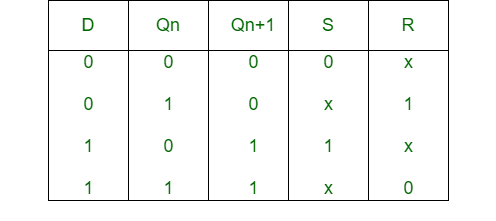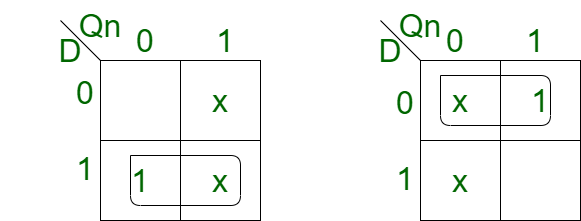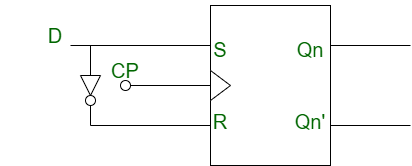# Conversion of S-R Flip-Flop into D Flip-Flop

• Difficulty Level : Basic
• Last Updated : 28 Apr, 2020

Prerequisite – Flip-flop

1. S-R Flip-Flop :
S-R flip-flop is similar to S-R latch expect clock signal and two AND gates. The circuit responds to the positive edge of clock pulse to the inputs S and R.

2. D Flip-Flop :
D Flip-Flop is a modified SR flip-flop which has an additional inverter. It prevents the inputs from becoming the same value.

Conversion of S-R Flip-Flop into D Flip-Flop :

• Step-1:
We construct the characteristic table of D flip-flop and excitation table of S-R flip-flop.• Step-2:
Using the K-map we find the boolean expression of S and R in terms of D.```S = D
R = D' ```
• Step-3:
We construct the circuit diagram of the conversion of S-R flip-flop into D flip-flop.My Personal Notes arrow_drop_up